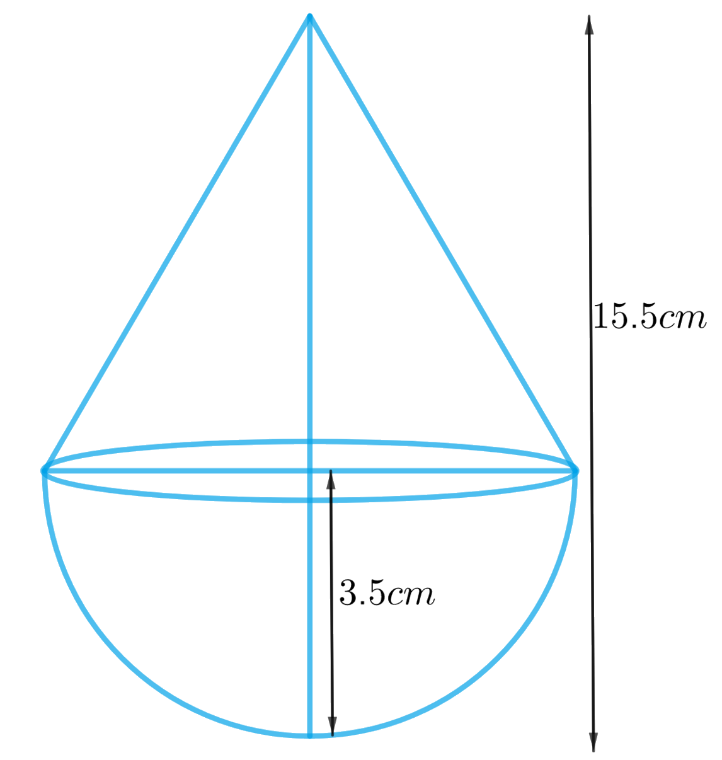# Ex.13.1 Q3 Surface Areas and Volumes Solution - NCERT Maths Class 10

Go back to  'Ex.13.1'

## Question

A toy is in the form of a cone of radius $$3.5\; \rm{cm}$$ mounted on a hemisphere of same radius. The total height of the toy is $$15.5 \;\rm{cm}$$. Find the total surface area of the toy.

## Text Solution

What is known?

The toy is in the form of a cone of radius $$=3.5\, \rm{cm}$$ mounted on a hemisphere with the same radius. The total height of the toy is $$15.5 \,\rm{cm}$$.

What is unknown?

The total surface area of the toy.

Reasoning:

We can create the figure of the toy as per given informationFrom the figure it’s clear that total surface area of the toy includes CSA of the cone and hemisphere.
Total surface area of the toy $$=$$ CSA of the hemisphere $$+$$ CSA of the cone

We will find the total area of the toy by using formulae;
CSA of the hemisphere$$= 2\pi {r^2}$$
where $$r$$ is the radius of the hemisphere

CSA of the cone $$= \pi rl$$
where $$r$$ and $$l$$ are the radius and slant height of the cone respectively.

Slant height of the cone, $$l = \sqrt {{r^2} + {h^2}}$$

Height of the cone, $$h =$$ total height of the toy $$–$$ height of the hemisphere

Steps:

Radius of the hemisphere, $$r = 3.5cm$$
Height of the hemisphere = radius of the hemisphere,  $$r = 3.5cm$$

Radius of the cone,  $$r = 3.5cm$$

Height of the cone $$=$$ Total height of the toy $$–$$ height of the hemisphere
$h = 15.5cm - 3.5cm = 12cm$

Slant height of the cone,  $$l = \sqrt {{r^2} + {h^2}}$$

\begin{align} l &= \sqrt {{r^2} + {h^2}} \\l &= \sqrt {{{\left( {3.5{cm}} \right)}^2} + {{\left( {12{cm}} \right)}^2}} \\l &= \sqrt {12.25{c{m^2}} + 144{c{m^2}}} \\l &= \sqrt {156.25{c{m^2}}} \\l &= 12.5{cm}\end{align}

Total surface area of the toy $$=$$ CSA of the hemisphere $$+$$ CSA of the cone

\begin{align}&= 2\pi {r^2} + \pi rl\\&= \pi r\left( {2r + l} \right)\\&= \frac{{22}}{7} \times 3.5{cm} \times \left( {2 \times 3.5{cm} + 12.5{cm}} \right)\\&= \frac{{22}}{7} \times \frac{7}{2}{cm} \times \left( {7{cm} + 12.5{cm}} \right)\\&= 11{cm} \times 19.5{cm}\\&= 214.5{c{m^2}}\end{align}

Learn from the best math teachers and top your exams

• Live one on one classroom and doubt clearing
• Practice worksheets in and after class for conceptual clarity
• Personalized curriculum to keep up with school